# Chapter 6.ppt

28 May 2023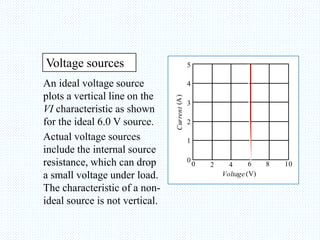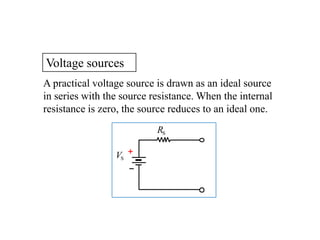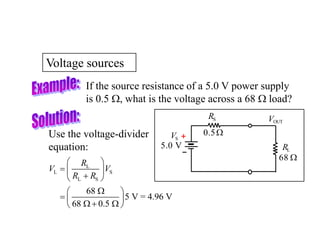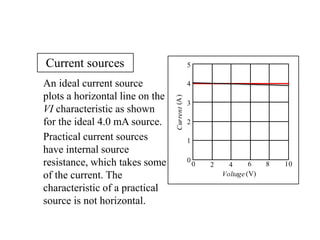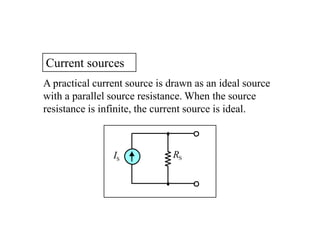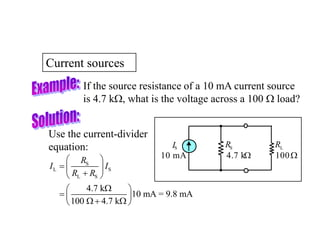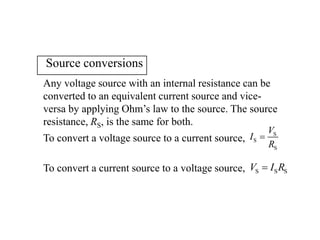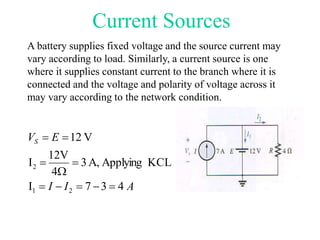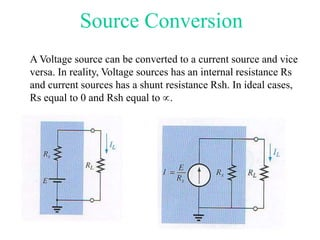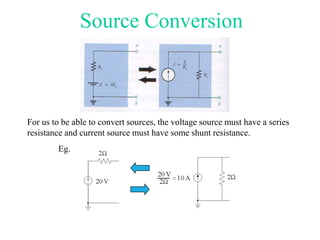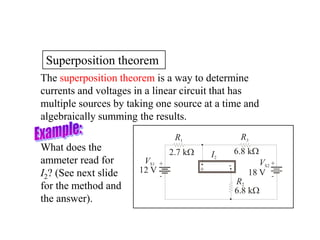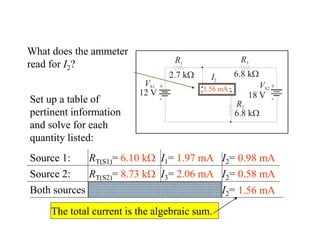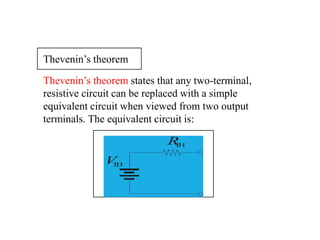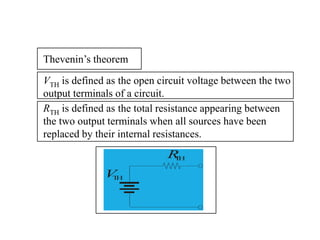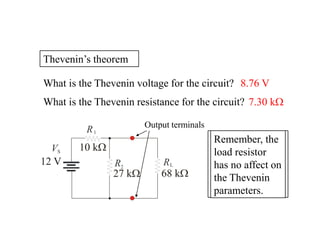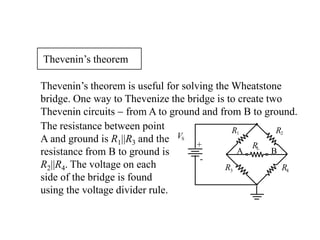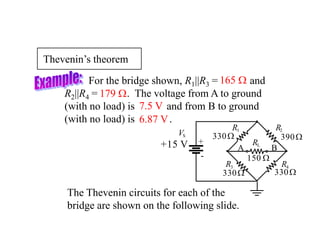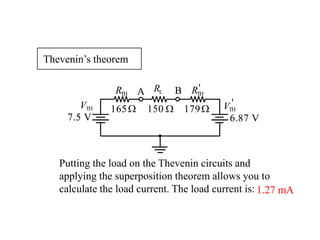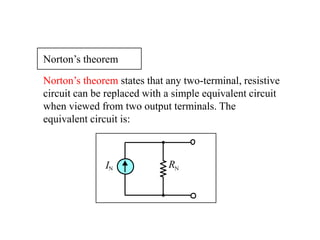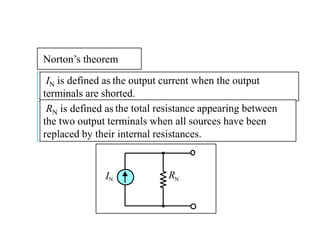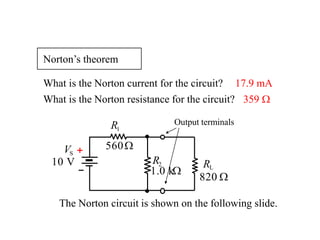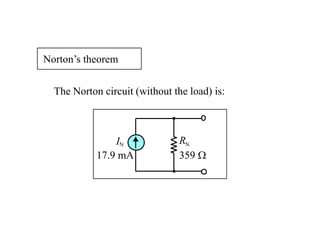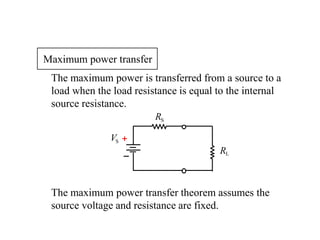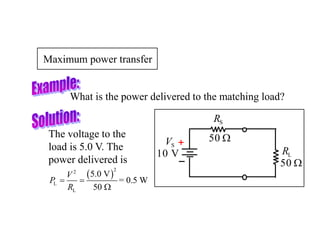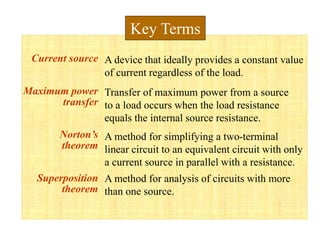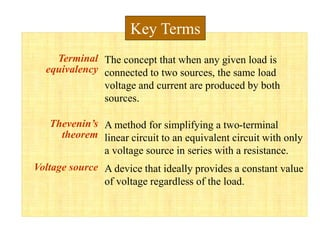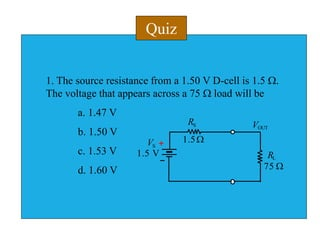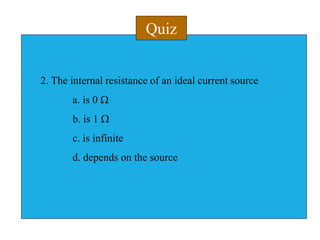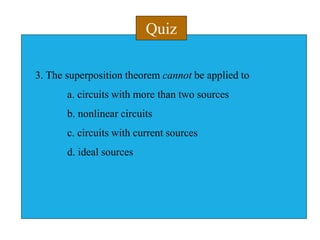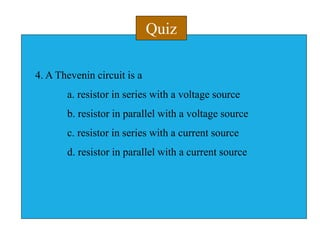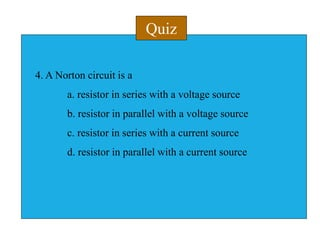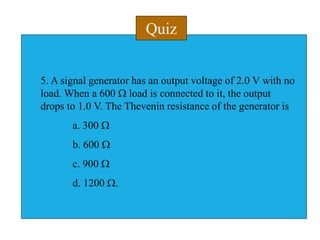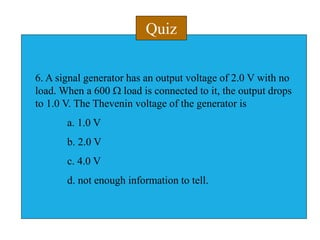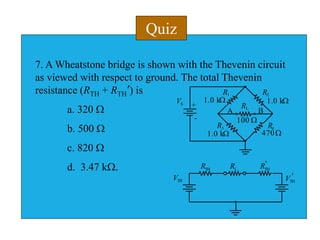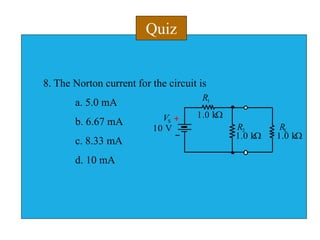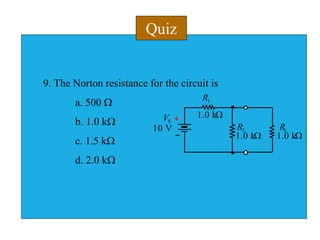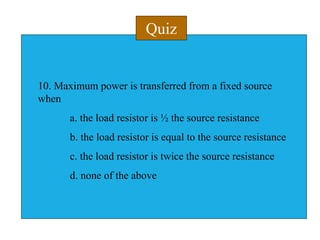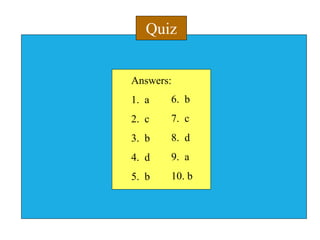1 sur 39

### Chapter 6.ppt

• 1. Principles of Electric Circuits - Floyd © Copyright 2006 Prentice-Hall Chapter 8
• 2. An ideal voltage source plots a vertical line on the VI characteristic as shown for the ideal 6.0 V source. Voltage sources Actual voltage sources include the internal source resistance, which can drop a small voltage under load. The characteristic of a non- ideal source is not vertical. Current (A) Voltage (V) 0 0 1 2 2 3 4 4 6 5 8 10
• 3. Voltage sources A practical voltage source is drawn as an ideal source in series with the source resistance. When the internal resistance is zero, the source reduces to an ideal one. RS VS +
• 4. Voltage sources If the source resistance of a 5.0 V power supply is 0.5 W, what is the voltage across a 68 W load? Use the voltage-divider equation: L L S L S 68 5 V = 4.96 V 68 0.5 R V V R R         W      W  W   RS RL VS VOUT + 5.0 V 0.5W 68 W
• 5. An ideal current source plots a horizontal line on the VI characteristic as shown for the ideal 4.0 mA source. Current sources Practical current sources have internal source resistance, which takes some of the current. The characteristic of a practical source is not horizontal. Current (A) Voltage (V) 0 0 1 2 2 3 4 4 6 5 8 10
• 6. A practical current source is drawn as an ideal source with a parallel source resistance. When the source resistance is infinite, the current source is ideal. Current sources RS IS
• 7. Current sources If the source resistance of a 10 mA current source is 4.7 kW, what is the voltage across a 100 W load? Use the current-divider equation: S L S L S 4.7 k 10 mA = 9.8 mA 100 4.7 k R I I R R         W      W  W   RS IS RL 100W 4.7 kW 10 mA
• 8. Source conversions Any voltage source with an internal resistance can be converted to an equivalent current source and vice- versa by applying Ohm’s law to the source. The source resistance, RS, is the same for both. To convert a voltage source to a current source, S S S V I R  S S S V I R  To convert a current source to a voltage source,
• 9. Current Sources A battery supplies fixed voltage and the source current may vary according to load. Similarly, a current source is one where it supplies constant current to the branch where it is connected and the voltage and polarity of voltage across it may vary according to the network condition. A I I E VS 4 3 7 I KCL Applying A, 3 4 12V I V 12 2 1 2       W   
• 10. Source Conversion A Voltage source can be converted to a current source and vice versa. In reality, Voltage sources has an internal resistance Rs and current sources has a shunt resistance Rsh. In ideal cases, Rs equal to 0 and Rsh equal to .
• 11. Source Conversion For us to be able to convert sources, the voltage source must have a series resistance and current source must have some shunt resistance. Eg.
• 12. Superposition theorem The superposition theorem is a way to determine currents and voltages in a linear circuit that has multiple sources by taking one source at a time and algebraically summing the results. What does the ammeter read for I2? (See next slide for the method and the answer). + - - + - + R1 R3 R2 I2 VS2 VS1 12 V 2.7 kW 6.8 kW 6.8 kW 18 V
• 13. 6.10 kW What does the ammeter read for I2? 1.97 mA 0.98 mA 8.73 kW 2.06 mA + - - + - + R1 R3 R2 I2 VS2 VS1 12 V 2.7 kW 6.8 kW 6.8 kW 18 V 0.58 mA 1.56 mA 1.56 mA Source 1: RT(S1)= I1= I2= Source 2: RT(S2)= I3= I2= Both sources I2= Set up a table of pertinent information and solve for each quantity listed: The total current is the algebraic sum.
• 14. Thevenin’s theorem states that any two-terminal, resistive circuit can be replaced with a simple equivalent circuit when viewed from two output terminals. The equivalent circuit is: Thevenin’s theorem V T H R T H
• 15. V T H R T H VTH is defined as Thevenin’s theorem RTH is defined as the open circuit voltage between the two output terminals of a circuit. the total resistance appearing between the two output terminals when all sources have been replaced by their internal resistances.
• 16. Thevenin’s theorem R R 1 R2 R2 L VS VS 12 V 10 kW 68 kW 27 kW Output terminals What is the Thevenin voltage for the circuit? 8.76 V What is the Thevenin resistance for the circuit? 7.30 kW Remember, the load resistor has no affect on the Thevenin parameters.
• 17. Thevenin’s theorem Thevenin’s theorem is useful for solving the Wheatstone bridge. One way to Thevenize the bridge is to create two Thevenin circuits  from A to ground and from B to ground. The resistance between point A and ground is R1||R3 and the resistance from B to ground is R2||R4. The voltage on each side of the bridge is found using the voltage divider rule. R3 R4 R2 RL R1 VS - + A B
• 18. Thevenin’s theorem For the bridge shown, R1||R3 = and R2||R4 = . The voltage from A to ground (with no load) is and from B to ground (with no load) is . The Thevenin circuits for each of the bridge are shown on the following slide. 165 W 179 W 7.5 V 6.87 V R3 R4 R2 RL R1 VS - + A B 330W 390W 330W 330W +15 V 150 W
• 19. Thevenin’s theorem Putting the load on the Thevenin circuits and applying the superposition theorem allows you to calculate the load current. The load current is: RL A B 150 W VTH VTH RTH RTH ' ' 165W 179W 7.5 V 6.87 V A B VTH VTH RTH RTH ' ' 165W 179W 7.5 V 6.87 V 1.27 mA
• 20. Norton’s theorem states that any two-terminal, resistive circuit can be replaced with a simple equivalent circuit when viewed from two output terminals. The equivalent circuit is: Norton’s theorem RN IN
• 21. Norton’s theorem the output current when the output terminals are shorted. the total resistance appearing between the two output terminals when all sources have been replaced by their internal resistances. IN is defined as RN is defined as RN IN
• 22. Norton’s theorem Output terminals What is the Norton current for the circuit? 17.9 mA What is the Norton resistance for the circuit? 359 W R2 R1 RL VS + 10 V 560W 820 W 1.0 kW The Norton circuit is shown on the following slide.
• 23. Norton’s theorem RN IN 17.9 mA 359 W The Norton circuit (without the load) is:
• 24. Maximum power transfer The maximum power is transferred from a source to a load when the load resistance is equal to the internal source resistance. The maximum power transfer theorem assumes the source voltage and resistance are fixed. RS RL VS +
• 25. Maximum power transfer What is the power delivered to the matching load? The voltage to the load is 5.0 V. The power delivered is RS RL VS + 50 W 50 W 10 V   2 2 L L 5.0 V = 0.5 W 50 V P R   W
• 26. Current source Maximum power transfer Norton’s theorem Superposition theorem Transfer of maximum power from a source to a load occurs when the load resistance equals the internal source resistance. A method for simplifying a two-terminal linear circuit to an equivalent circuit with only a current source in parallel with a resistance. A device that ideally provides a constant value of current regardless of the load. Key Terms A method for analysis of circuits with more than one source.
• 27. Terminal equivalency Thevenin’s theorem Voltage source A method for simplifying a two-terminal linear circuit to an equivalent circuit with only a voltage source in series with a resistance. The concept that when any given load is connected to two sources, the same load voltage and current are produced by both sources. Key Terms A device that ideally provides a constant value of voltage regardless of the load.
• 28. Quiz 1. The source resistance from a 1.50 V D-cell is 1.5 W. The voltage that appears across a 75 W load will be a. 1.47 V b. 1.50 V c. 1.53 V d. 1.60 V RS RL VS VOUT + 1.5 V 1.5W 75 W
• 29. Quiz 2. The internal resistance of an ideal current source a. is 0 W b. is 1 W c. is infinite d. depends on the source
• 30. Quiz 3. The superposition theorem cannot be applied to a. circuits with more than two sources b. nonlinear circuits c. circuits with current sources d. ideal sources
• 31. Quiz 4. A Thevenin circuit is a a. resistor in series with a voltage source b. resistor in parallel with a voltage source c. resistor in series with a current source d. resistor in parallel with a current source
• 32. Quiz 4. A Norton circuit is a a. resistor in series with a voltage source b. resistor in parallel with a voltage source c. resistor in series with a current source d. resistor in parallel with a current source
• 33. Quiz 5. A signal generator has an output voltage of 2.0 V with no load. When a 600 W load is connected to it, the output drops to 1.0 V. The Thevenin resistance of the generator is a. 300 W b. 600 W c. 900 W d. 1200 W.
• 34. Quiz 6. A signal generator has an output voltage of 2.0 V with no load. When a 600 W load is connected to it, the output drops to 1.0 V. The Thevenin voltage of the generator is a. 1.0 V b. 2.0 V c. 4.0 V d. not enough information to tell.
• 35. Quiz 7. A Wheatstone bridge is shown with the Thevenin circuit as viewed with respect to ground. The total Thevenin resistance (RTH + RTH’) is a. 320 W b. 500 W c. 820 W d. 3.47 kW. R3 R4 R2 RL R1 VS - + A B 1.0 kW 1.0 kW 1.0 kW 470W 100 W VTH VTH RTH RL RTH ' '
• 36. Quiz 8. The Norton current for the circuit is a. 5.0 mA b. 6.67 mA c. 8.33 mA d. 10 mA R2 R1 RL VS + 10 V 1.0 kW 1.0 kW 1.0 kW
• 37. Quiz 9. The Norton resistance for the circuit is a. 500 W b. 1.0 kW c. 1.5 kW d. 2.0 kW R2 R1 RL VS + 10 V 1.0 kW 1.0 kW 1.0 kW
• 38. Quiz 10. Maximum power is transferred from a fixed source when a. the load resistor is ½ the source resistance b. the load resistor is equal to the source resistance c. the load resistor is twice the source resistance d. none of the above
• 39. Quiz Answers: 1. a 2. c 3. b 4. d 5. b 6. b 7. c 8. d 9. a 10. b